# Texas Go Math Grade 3 Lesson 6.5 Answer Key Commutative Property of Multiplication

Refer to our Texas Go Math Grade 3 Answer Key Pdf to score good marks in the exams. Test yourself by practicing the problems from Texas Go Math Grade 3 Lesson 6.5 Answer Key Commutative Property of Multiplication.

## Texas Go Math Grade 3 Lesson 6.5 Answer Key Commutative Property of Multiplication

Essential Question
How can you use the Commutative Property of Multiplication to find products?
The commutative property of multiplication also applies when you multiply more than two numbers.
When we have more than two numbers, we
can switch around the numbers any which way we want.
ab = b a; a b = b a.

Unlock the Problem
Dave works at the Bird Store. He arranges 15 boxes of birdseed in rows on the shelf. What are two ways he can arrange the boxes in equal rows?
3 x 5 = 15
5 x 3 = 15
Explanation:
Dave arranges 15 boxes of birdseed in rows on the shelf.
The following are the two ways he can arrange the boxes in equal rows
3 x 5 = 15
5 x 3 = 15

Activity
Make an array.
Materials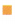square tilesMath Board

Arrange 15 tiles in 5 equal rows. Draw a quick picture of your array.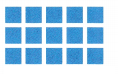5 x 3 = 15
Explanation:
15 tiles are arranged i array as
5 rows of 3.

How many tiles are in each row? ___
5 tiles
What multiplication sentence does your array show? ___
5 x 3 = 15

Suppose Dave arranges the boxes in 3 equal rows. Draw a quick picture of your array.3 x 5 = 15
Explanation:
15 tiles are arranged in array as
3 rows of 5.

How many tiles are in each row? ___

What multiplication sentence does your array show? ________
Multiply 3 x 5 = 15
So, two ways Dave can arrange the 15 boxes are in 5 rows of 3 or in 3 rows of 5.

Math Talk
Mathematical Processes
Why do 5 rows of 3 and 3 rows of 5 both equal the same number?
Multiplication Property The Commutative Property of Multiplication states that when you change the order of the factors, the product stays the same. You can think of it as the Order Property of Multiplication.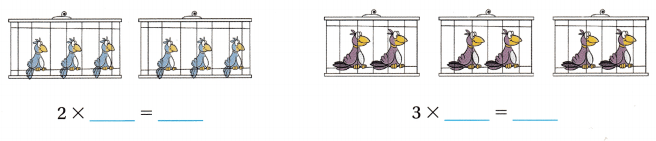So, 2 × __ = 3 × __.
So, 2 × 3 = 3 × 2

Math Idea
Facts that show the Commutative Property of Multiplication have the same factors in a different order.
2 × 3 = 6 and 3 × 2 = 6

Share and Show

Question 1.
Write a multiplication sentence for the array.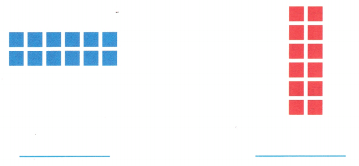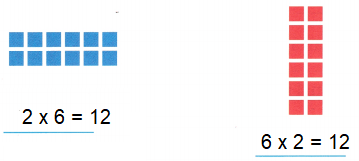Explanation:
The commutative property of multiplication states that for any a x b = b x a
So, 2 x 6 = 6 x 2 = 12

Math Talk
Mathematical Processes
Explain what the factor 2 means in each multiplication sentence.
In a multiplication sentence, the two numbers either side of the multiplication sign are called factors.
The number after the equals sign is called the multiple.
Explanation:
For example:
If you write the two smaller numbers the other way around as 5 × 3 = 15 instead of 3 × 5 =15,
It would mean 5 lots of 3.

Write a multiplication sentence for the model. Then use the Commutative Property of Multiplication to write a related multiplication sentence.

Go Math Grade 3 Lesson 6.5 Commutative Property of Multiplication Question 2.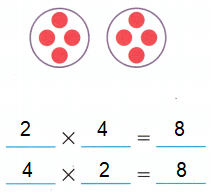Explanation:
The commutative property of multiplication states that when you change the order of the factors,
the product stays the same a x b = b x a.

Question 3.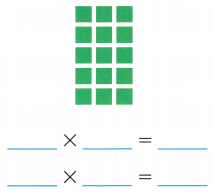Explanation:
The commutative property of multiplication states that when you change the order of the factors,
the product stays the same a x b = b x a.

Question 4.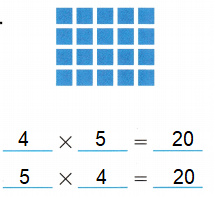Explanation:
The commutative property of multiplication states that when you change the order of the factors,
the product stays the same a x b = b x a.

Problem Solving

Question 5.
Write Math How is the Commutative Property of Multiplication like the Commutative Property of Addition?
The commutative property of addition states that for any a and b,
a + b = b = a,
similarly;
The commutative property of multiplication states that for any a x b = b x a
Explanation:
The commutative property essentially means that the order in which we perform a single addition or multiplication does not matter.

H.O.T. Algebra Write the unknown factor.

Question 6.
3 × 7 = __ × 3
Explanation:
Commutative Property of Multiplication
x  x  y  = y  x  x
3 x 7 = 7 x 3 = 21

4 × 5 = 10 × ___
Explanation:
Commutative Property of Multiplication
x  x  y  = y  x  x
4 x 5 = 20 = 10 x 2

Question 8.
3 × 6 = ___ × 9
Explanation:
Commutative Property of Multiplication
x  x  y  = y  x  x
3 x 6 = 18 = 2 x 9

Question 9.
6 × __ = 4 × 9
Explanation:
Commutative Property of Multiplication
x  x  y  = y  x  x
6 x 6 = 36 = 4 x 9

Question 10.
__ × 8 = 4 × 6
Explanation:
Commutative Property of Multiplication
x  x  y  = y  x  x
3 x 8 = 24 = 4 x 6

Question 11.
5 × 8 = 8 × ___
Commutative Property of Multiplication
x  x  y  = y  x  x
5 x 8 = 40 = 8 x 5

Problem Solving

Question 12.
Jenna used pinecones to make 18 peanut butter bird feeders. She hung the same number of feeders in each of 6 trees. Draw an array to show how many feeders she put in each tree.
She put ___ bird feeders in each tree.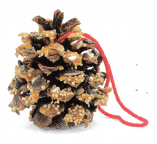6 x 3 = 18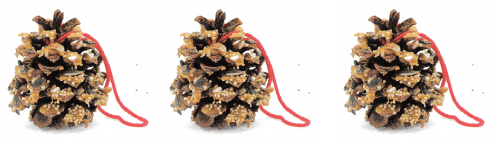Explanation:
Jenna used pinecones to make 18 peanut butter bird feeders.
She hung the same number of feeders in each of 6 trees.
6 x 3 = 18
She put 3 bird feeders in each tree.

H.O.T. Multi-Step There are 4 rows of 6 bird stickers in Don’s sticker album. There are 7 rows of 5 bird stickers in Lindsey’s album. How many bird stickers do they have in all?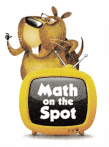59 bird stickers.
Explanation:
There are 4 rows of 6 bird stickers in Don’s sticker album.
There are 7 rows of 5 bird stickers in Lindsey’s album.
Number of bird stickers they have in all
4 x 6 + 7 x 5 = 24 + 35 = 59

Question 14.
Write Math Write two different word problems about 12 birds to show 2 × 6 and 6 × 2. Solve each problem.
Word problem:
Jenna saw 6 birds sitting on 2 branches, how many birds were on the tree?
2 x 6 = 12
Sabastian saw 2 birds sitting on one branch, a total of 6 branches are there t that tree. total how many birds are there?
6 x 2 =12

Fill in the bubble for the correct answer choice.
You can use objects or models to solve.

Question 15.
Sebastian has 4 equal rows of action figures. There are 5 action figures in each row. One way to find the number of action figures is to multiply 4 × 5. What is another way to find the number of action figures?
(C) multiply 5 × 4
(D) multiply 6 × 5
Option (C)
Explanation:
Sebastian has 4 equal rows of action figures.
There are 5 action figures in each row.
One way to find the number of action figures is to multiply 4 × 5.
Another way to find the number of action figures is to multiply 5 x 4.

Question 16.
Use Tools Rachel has 24 stamps. She arranges them in 3 rows with 8 stamps in each row. What is another way she could arrange the 24 stamps?
(A) 2 rows of 8 stamps
(B) 8 rows of 3 stamps
(C) 8 rows of 4 stamps
(D) 6 rows of 3 stamps
Option (B)
Explanation:
Rachel has 24 stamps.
She arranges them in 3 rows with 8 stamps in each row.
Another way she could arrange the 24 stamps
8 rows of 3 stamps.

Multi-Step Mr. Diaz sets out 6 rows of glasses with 3 glasses in each row. Mrs. Diaz sets out 3 rows of glasses with 6 glasses in each row. How many glasses do Mr. and Mrs. Diaz set out in all?
(A) 36
(B) 54
(C) 18
(D) 24
Option (D)
Explanation:
Mr. Diaz sets out 6 rows of glasses with 3 glasses in each row.
Mrs. Diaz sets out 3 rows of glasses with 6 glasses in each row.
Number of glasses Mr. and Mrs. Diaz set out in all
6 x 3 + 3 x 6 = 12 + 12 = 24

Texas Test prep

Question 18.
Communicate Which is an example of the Commutative Property of Multiplication?
(A) 2 × 6 = 3 × 4
(B) 4 × 1 = 4 × 1
(C) 2 × 4 = 4 × 2
(D) 2 + 3 = 3 × 2
Option (C)
Explanation:
The commutative property of multiplication also applies when you multiply more than two numbers.
When we have more than two numbers, we
can switch around the numbers any way we want.
ab = b a; a b = b a.

### Texas Go Math Grade 3 Lesson 6.5 Homework and Practice Answer Key

Write a multiplication sentence for each model. Then use the Commutative Property of Multiplication to write a related multiplication sentence.

Question 1.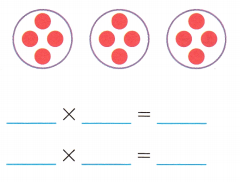3 x 4 = 12
4 x 3 = 12
Explanation:
In the Commutative Property, more than two numbers can switch around the numbers any way we want.
ab = b a; a b = b a.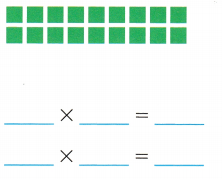2 x 9 = 18
9 x 2 = 18
Explanation:
In the Commutative Property more than two numbers can switch around the numbers any which way we want.
ab = b a; a b = b a.

Question 3.
Josh made 14 potholders. He put an equal number of potholders in each of 2 boxes. Write two related multiplication sentences to show how many potholders Josh made.
___ × ___ = ___
___ × ___ = ___
7 x 2 = 14
2 x 7 = 14
Explanation:
He put an equal number of potholders in each of 2 boxes.
Two related multiplication sentences to show potholders
7 x 2 = 14; 2 x 7 = 14

Question 4.
Lu Chen has 21 rocks in a collection. He puts the same number of rocks in each of 3 boxes. Draw an array to show how many rocks are in each box.
Explanation:
Lu Chen has 21 rocks in a collection.
He puts the same number of rocks in each of 3 boxes.
Multiply 3 x 7 =21

Problem Solving

Question 5.
Serena makes 5 belts. Each belt has 4 beads. Serena writes 5 × 4 = 20 to show the number of beads. Write another multiplication sentence to show the number of beads.
Multiply 4 x 5
Explanation:
Serena makes 5 belts. Each belt has 4 beads.
Serena writes 5 × 4 = 20 to show the number of beads.
Another multiplication sentence to show the number of beads.
4 x 5 = 20

Go Math Lesson 6.5 Commutative Property of Multiplication 3rd Grade Question 6.
Dikembe has 3 packs of 10 baseball cards. He writes 3 × 10 = 30 to show the number of cards. Write another multiplication sentence to show the number of baseball cards.
Multiply 10 x 3
Dikembe has 3 packs of 10 baseball cards.
He writes 3 × 10 = 30 to show the number of cards.
Another multiplication sentence to show the number of baseball cards.
10 x 3 = 30

Lesson Check

Texas Test Prep

Question 7.
There are 7 vases with 5 flowers in each vase. Gina multiplies 7 × 5 to find the number of flowers. What is another way to find the number of flowers?
(C) multiply 5 × 7
(D) multiply 7 × 7
Option (C)
There are 7 vases with 5 flowers in each vase.
Gina multiplies 7 × 5 to find the number of flowers.
Another way to find the number of flowers
5 vases with 7 flowers
5 x 7 = 35

Question 8.
A photo album has 8 pages with 6 photos on each page. Cory multiplies 8 × 6 to find the number of photos. What is another way to find the number of photos?
(B) multiply 6 × 8
(D) multiply 6 × 6
Option (B)
Explanation:
A photo album has 8 pages with 6 photos on each page.
8 x 6 = 48
Another way to find the number of photos
6 rows of 8
6 x 8 = 48

Lesson 6.5 Commutative Property 3rd Grade Go Math Question 9.
Demi has 36 toy cars. She puts them in 4 rows with 9 in each row. What is another way Demi can arrange the toy cars to show the Commutative Property of Multiplication?
(A) 6 rows of 6
(B) 3 rows of 12
(C) 9 rows of 4
(D) 2 rows of 18
Option (A)
Explanation:
Demi has 36 toy cars.
She puts them in 4 rows with 9 in each row.
4 x 9 = 36
Another way Demi can arrange the toy cars to show the Commutative Property of Multiplication
6 rows of 6
6 x 6 = 36

Question 10.
Risa makes this model.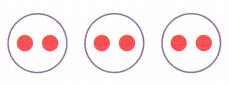Which of the following can Risa write to show the Commutative Property of Multiplication?
(A) 3 × 2 = 2 × 3
(B) 2 × 2 = 3 × 3
(C) 3 × 2 = 1 × 6
(D) 3 × 2 = 1 × 6
Option (A)
Explanation:
In the Commutative Property, more than two numbers can switch around the numbers any way we want.
ab = b a; a b = b a.
3 × 2 = 2 × 3

Multi-Step Juan puts 3 rows of 5 stickers in a book. Then he puts 5 rows of 3 stickers on the next page. How many stickers does Juan put in the book?
(A) 15
(B) 16
(C) 30
(D) 25
Option (C)
Explanation:
Juan puts 3 rows of 5 stickers in a book.
Then he puts 5 rows of 3 stickers on the next page.
Number of stickers Juan put in the book
3 x 5 = 15 + 5 x 3 = 15
15 + 15 = 30

Question 12.
Multi-Step One classroom has 4 rows of 5 chairs. Another classroom has 5 rows of 4 chairs. How many chairs are there in both classrooms?
(A) 20
(B) 19
(C) 25
(D) 40